# Logical Equivalences

Tom Kelliher, MA 190

Feb. 1, 2008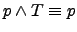Identity laws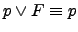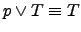Domination laws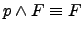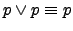Idempotent laws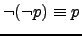Double negation lawCommutative laws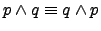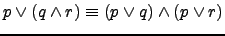Distributive laws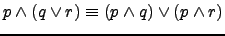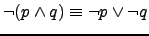De Morgan's laws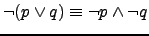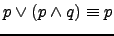Absorption laws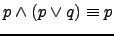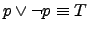Negation laws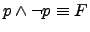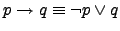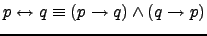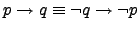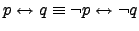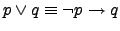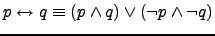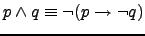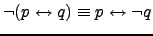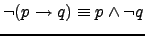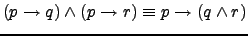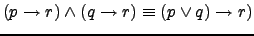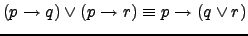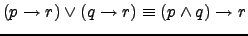## Exercises

1. (5) Verify using a truth table:.

2. (7 a, c) Use De Morgan's laws to find the negation of
1. Jan is rich and happy.
2. Mei walks or takes the bus to class.

3. (9 a, d) Use truth tables to show that the following are tautologies
1.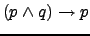2.4. (11 a, d) Use a series of logical equivalences to show that the previous two compound propositions are tautologies.

5. (15) Determine whether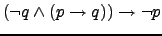is a tautology.

6. (23, 25) Show that the following compound propositions are logically equivalent by showing that both sides are true, or that both sides are false, for exactly the same combinations of truth values of the propositional variables in these expressions (whichever is easier).
1.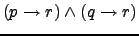and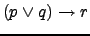.
2.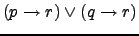and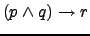.

Thomas P. Kelliher 2008-01-30
Tom Kelliher